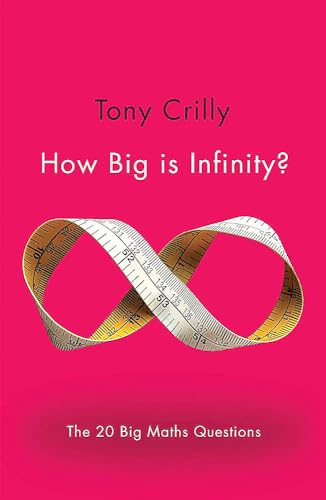Free Shipping on all orders in Australia
Over 7 million books in stock
Proud to be B-Corp# How Big is Infinity? by Tony Crilly

Condition - Very Good
\$13.69
Only 3 left

## Summary

Answers to 20 of the most mindbending and perplexing mathematical questions.

## How Big is Infinity? Summary

### How Big is Infinity?: The 20 Big Maths Questions by Tony Crilly

What are the strangest numbers? Where do numbers come from? Can maths guarantee riches? Why are three dimensions not enough? Can a butterfly's wings really cause a hurricane? Can maths predict the future? In How Big is Infinity?, acclaimed writer Tony Crilly distills the wisdom of some of the greatest minds in history to help provide answers some of the most perplexing, stimulating and surprising questions in mathematics.

Tony Crilly is a Reader in Mathematical Sciences at Middlesex University, having previously taught at the University of Michigan, the City University in Hong Kong and the Open University. His principal research interest is the history of mathematics, and he has written and edited many works on fractals, chaos and computing. He is the author of the acclaimed biography of the English mathematician Arthur Cayley and the hugely successful 50 Mathematical Ideas You Really Need to Know.

Introduction. What is mathematics for? - An introduction to purposes and prospects. Where do numbers come from? - From notches on bones to hexadecimals. Why are primes the atoms of mathematics? - Building blocks and the fundamental theorem of arithmetic. Which are the strangest numbers? - Real, irrational and transcendental numbers. Are imaginary numbers truly imaginary? - From the imaginary 'I' to octonions. How big is infinity? - Set theory and the infinity revolution. Where do parallel lines meet? - The birth of new geometries. What is the mathematics of the universe? - The Calculus miracle. Are statistics lies? - Data, proof and 'damned lies'. Can mathematics guarantee riches? - Uncertainty, chance and probability theory. Is there a formula for everything? - Mathematical recipes and the search for knowledge. Why are three dimensions not enough? - Higher dimensions, monster curves and fractals. Can a butterfly's wings really cause a hurricane? - Chaos theory, weather equations and strange attractors. Can we create an unbreakable code? - Ciphers, the Enigma machine and quantum computers. Is mathematics beautiful? - Music, art, golden numbers and the Fibonacci sequence. Can mathematics predict the future? - Mathematical models, simulations and game theory. What shape is the universe? - Topology, manifolds and the Poincare conjecture. What is symmetry? - Patterns, dualities and the fundamental nature of reality. Is mathematics true? - From Plato's reality to Godel's incompleteness theorems. Is there anything left to solve? - The great unsolved problems and the future of mathematics. Glossary. Index.

GOR006003548
9781782069485
1782069488
How Big is Infinity?: The 20 Big Maths Questions by Tony Crilly
Used - Very Good
Paperback
Quercus Publishing
2014-07-03
304
N/A
Book picture is for illustrative purposes only, actual binding, cover or edition may vary.
This is a used book - there is no escaping the fact it has been read by someone else and it will show signs of wear and previous use. Overall we expect it to be in very good condition, but if you are not entirely satisfied please get in touch with us